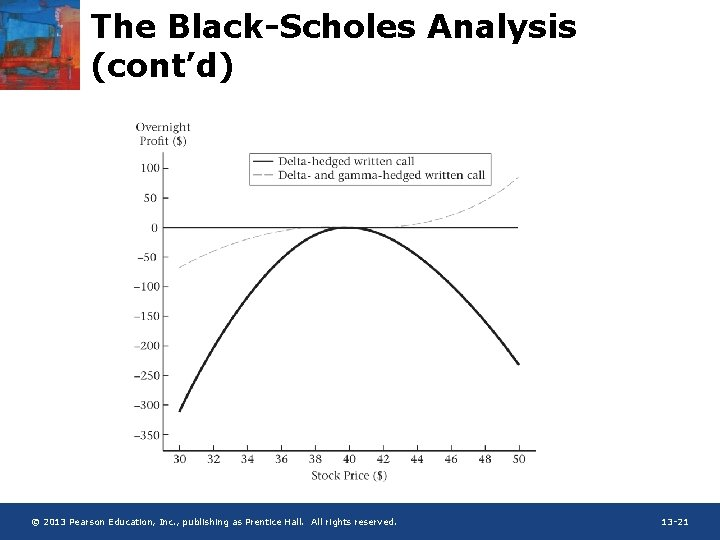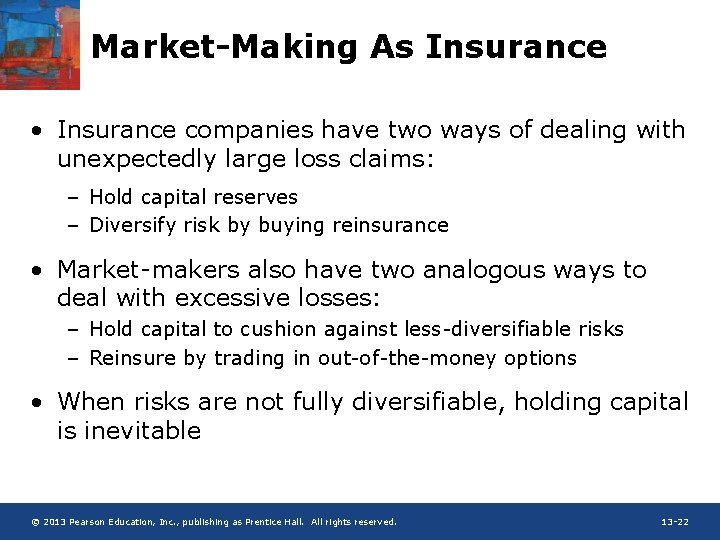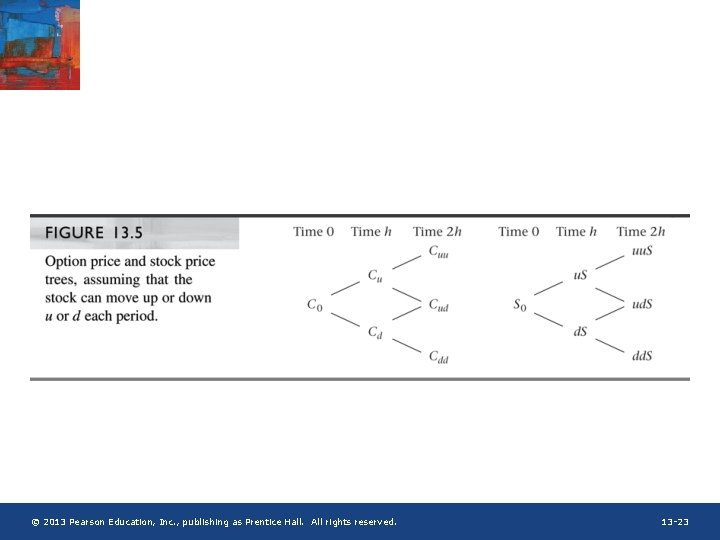# Chapter 13 MarketMaking and DeltaHedging What Do Market

• Slides: 23Chapter 13 Market-Making and Delta-Hedging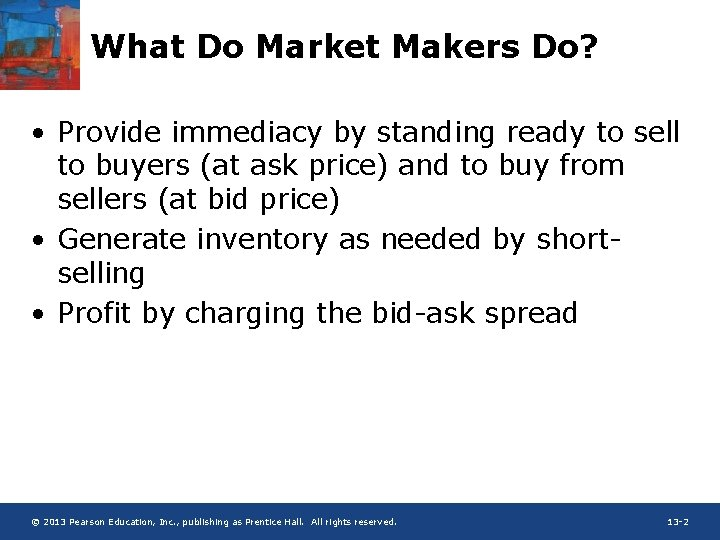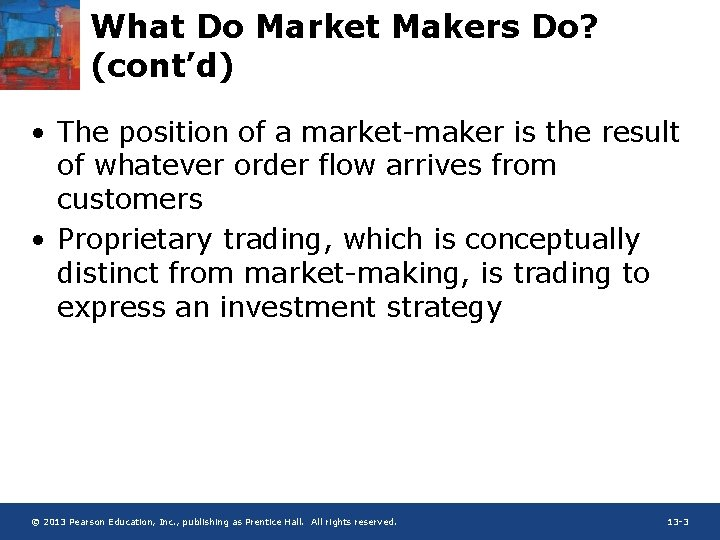What Do Market Makers Do? (cont’d) • The position of a market-maker is the result of whatever order flow arrives from customers • Proprietary trading, which is conceptually distinct from market-making, is trading to express an investment strategy © 2013 Pearson Education, Inc. , publishing as Prentice Hall. All rights reserved. 13 -3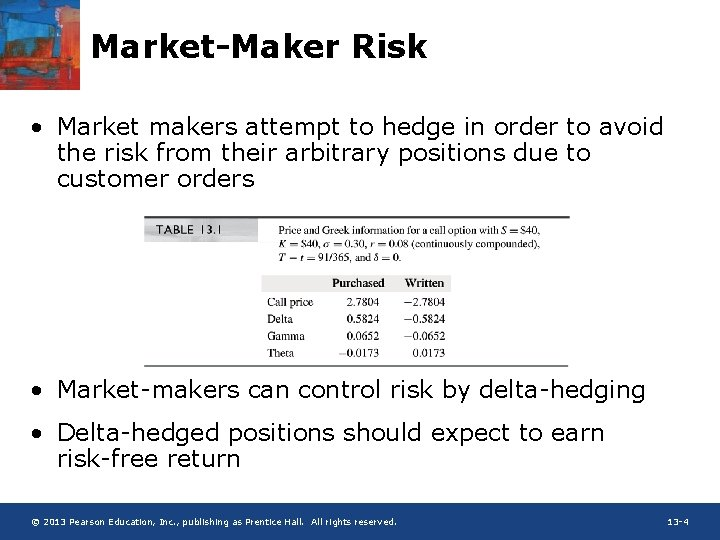Market-Maker Risk • Market makers attempt to hedge in order to avoid the risk from their arbitrary positions due to customer orders • Market-makers can control risk by delta-hedging • Delta-hedged positions should expect to earn risk-free return © 2013 Pearson Education, Inc. , publishing as Prentice Hall. All rights reserved. 13 -4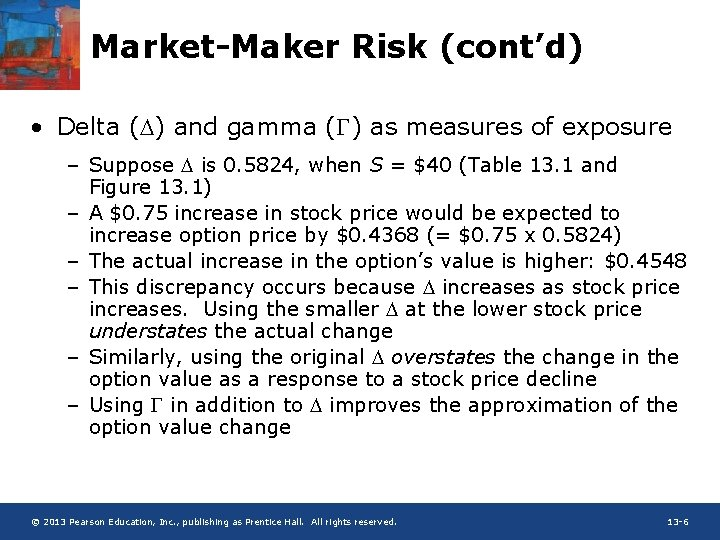Market-Maker Risk (cont’d) • Delta (D) and gamma ( ) as measures of exposure – Suppose D is 0. 5824, when S = \$40 (Table 13. 1 and Figure 13. 1) – A \$0. 75 increase in stock price would be expected to increase option price by \$0. 4368 (= \$0. 75 x 0. 5824) – The actual increase in the option’s value is higher: \$0. 4548 – This discrepancy occurs because D increases as stock price increases. Using the smaller D at the lower stock price understates the actual change – Similarly, using the original D overstates the change in the option value as a response to a stock price decline – Using in addition to D improves the approximation of the option value change © 2013 Pearson Education, Inc. , publishing as Prentice Hall. All rights reserved. 13 -6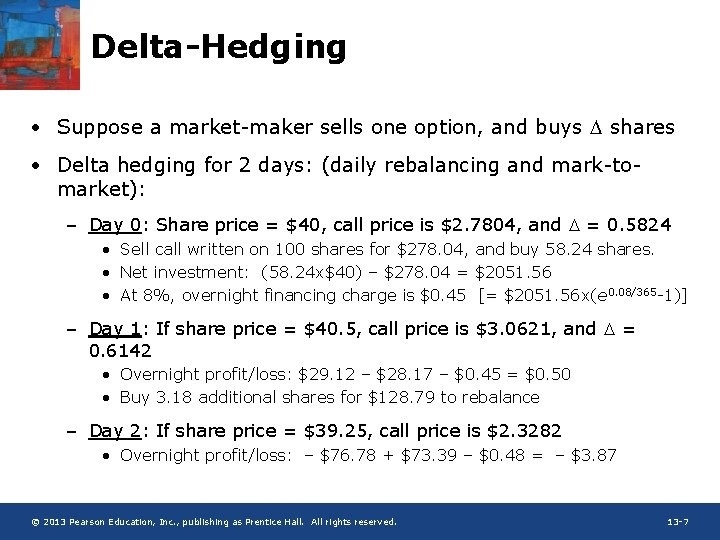Delta-Hedging • Suppose a market-maker sells one option, and buys D shares • Delta hedging for 2 days: (daily rebalancing and mark-tomarket): – Day 0: Share price = \$40, call price is \$2. 7804, and D = 0. 5824 • Sell call written on 100 shares for \$278. 04, and buy 58. 24 shares. • Net investment: (58. 24 x\$40) – \$278. 04 = \$2051. 56 • At 8%, overnight financing charge is \$0. 45 [= \$2051. 56 x(e 0. 08/365 -1)] – Day 1: If share price = \$40. 5, call price is \$3. 0621, and D = 0. 6142 • Overnight profit/loss: \$29. 12 – \$28. 17 – \$0. 45 = \$0. 50 • Buy 3. 18 additional shares for \$128. 79 to rebalance – Day 2: If share price = \$39. 25, call price is \$2. 3282 • Overnight profit/loss: – \$76. 78 + \$73. 39 – \$0. 48 = – \$3. 87 © 2013 Pearson Education, Inc. , publishing as Prentice Hall. All rights reserved. 13 -7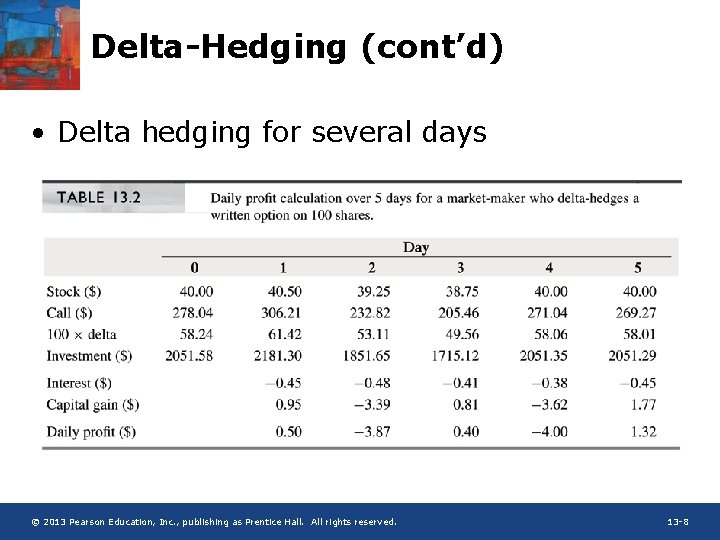Delta-Hedging (cont’d) • Delta hedging for several days © 2013 Pearson Education, Inc. , publishing as Prentice Hall. All rights reserved. 13 -8Delta-Hedging (cont’d) • Delta hedging for several days (cont. ) – : For large decreases in stock price, D decreases, and the option increases in value slower than the loss in stock value. For large increases in stock price D increases, and the option decreases in value faster than the gain in stock value. In both cases the net loss increases and the market -maker loses money. For small moves in the stock price, the market-maker makes money. – q : If a day passes with no change in the stock price, the option becomes cheaper. Since the option position is short, this time decay increases the profits of the marketmaker. – Interest cost: In creating the hedge, the market-maker purchases the stock with borrowed funds. The carrying cost of the stock position decreases the profits of the market-maker. © 2013 Pearson Education, Inc. , publishing as Prentice Hall. All rights reserved. 13 -9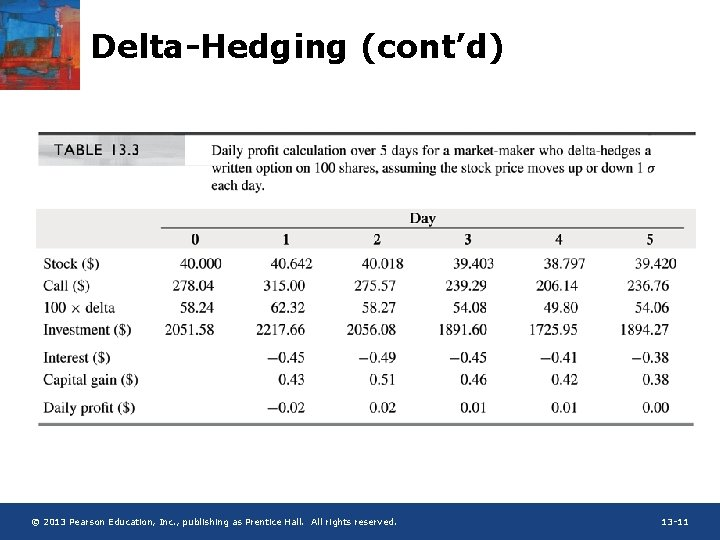Mathematics of ∆-Hedging • D- approximations – Recall the under (over) estimation of the new option value using D alone when stock price moved up (down) by e. (e = St+h – St) – Using the D- approximation the accuracy can be improved a lot – Example 13. 1: S: \$40. 75, C: \$2. 7804 \$3. 2352, : 0. 0652 • Using D approximation C(\$40. 75) = C(\$40) + 0. 75 x 0. 5824 = \$3. 2172 • Using D- approximation C(\$40. 75) = C(\$40) + 0. 75 x 0. 5824 + 0. 5 x 0. 752 x 0. 0652 = \$3. 2355 • Similarly, for a stock price decline to \$39. 25, the true option price is \$2. 3622. The D approximation gives \$23436, and the D- approximation gives \$2. 3619. © 2013 Pearson Education, Inc. , publishing as Prentice Hall. All rights reserved. 13 -12Mathematics of ∆-Hedging (cont’d) • D- approximation (cont’d) © 2013 Pearson Education, Inc. , publishing as Prentice Hall. All rights reserved. 13 -13Mathematics of ∆-Hedging (cont’d) • q: Accounting for time © 2013 Pearson Education, Inc. , publishing as Prentice Hall. All rights reserved. 13 -14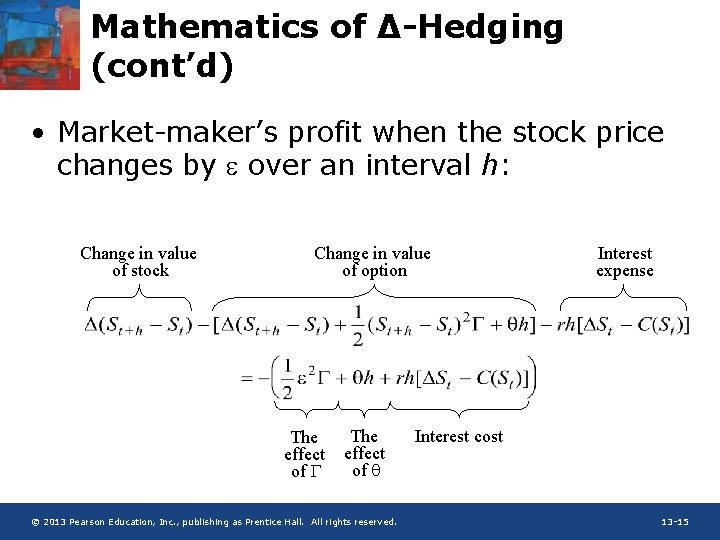Mathematics of ∆-Hedging (cont’d) • Market-maker’s profit when the stock price changes by e over an interval h: Change in value of stock Change in value of option The effect of q © 2013 Pearson Education, Inc. , publishing as Prentice Hall. All rights reserved. Interest expense Interest cost 13 -15Mathematics of ∆-Hedging (cont’d) • Note that D, and q are computed at t • For simplicity, the subscript “t” is omitted in the above equation © 2013 Pearson Education, Inc. , publishing as Prentice Hall. All rights reserved. 13 -16Mathematics of ∆-Hedging (cont’d) • If s is measured annually, then a one-standarddeviation move e over a period of length h is Therefore, © 2013 Pearson Education, Inc. , publishing as Prentice Hall. All rights reserved. 13 -17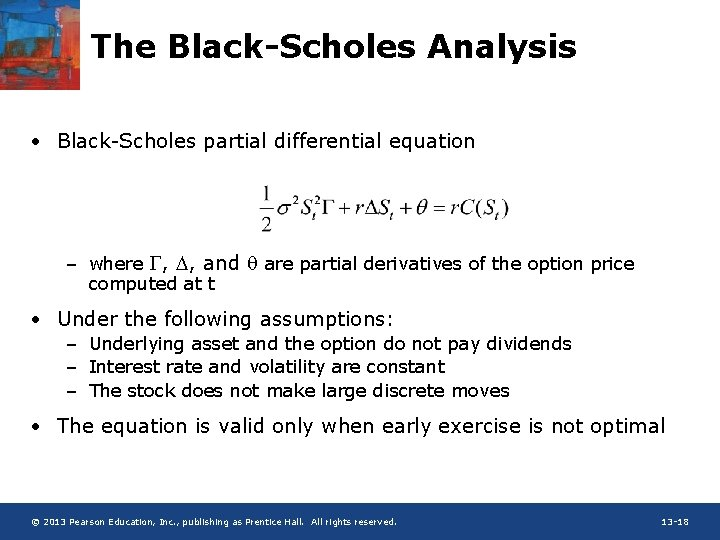The Black-Scholes Analysis • Black-Scholes partial differential equation – where , D, and q are partial derivatives of the option price computed at t • Under the following assumptions: – Underlying asset and the option do not pay dividends – Interest rate and volatility are constant – The stock does not make large discrete moves • The equation is valid only when early exercise is not optimal © 2013 Pearson Education, Inc. , publishing as Prentice Hall. All rights reserved. 13 -18The Black-Scholes Analysis (cont’d) • Advantage of frequent re-hedging – Varhourly = 1/24 x Vardaily – By hedging hourly instead of daily, total return variance is reduced by a factor of 24 – The more frequent hedger benefits from diversification over time • Three ways for protecting against extreme price moves – Adopt a -neutral position by using options to hedge – Augment the portfolio by buying deep-out-of-the-money puts and calls as insurance – Use static option replication according to put-call parity to form a - and D-neutral hedge © 2013 Pearson Education, Inc. , publishing as Prentice Hall. All rights reserved. 13 -19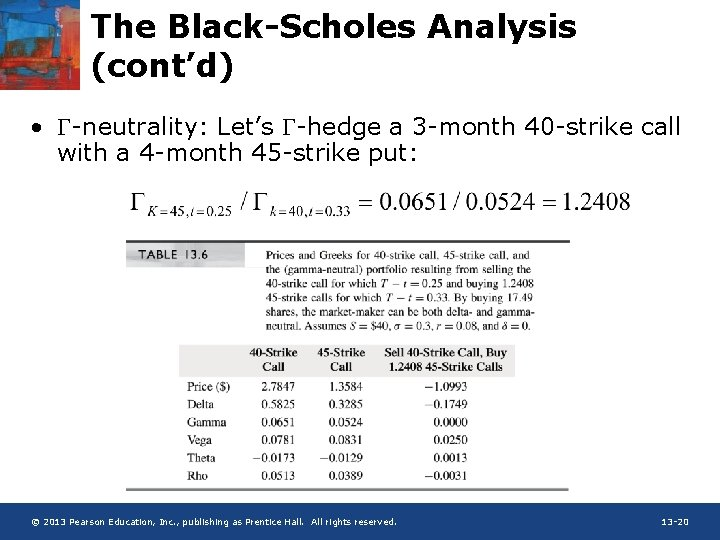The Black-Scholes Analysis (cont’d) • -neutrality: Let’s -hedge a 3 -month 40 -strike call with a 4 -month 45 -strike put: © 2013 Pearson Education, Inc. , publishing as Prentice Hall. All rights reserved. 13 -20